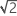# Using the number of distinct categories

## What is the number of distinct categories?

The number of distinct categories is a metric that is used in gage R&R studies to identify a measurement system's ability to detect a difference in the measured characteristic. The number of distinct categories represents the number of non-overlapping confidence intervals that span the range of product variation. The number of distinct categories also represents the number of groups within your process data that your measurement system can discern.

Minitab calculates the number of distinct categories by dividing the standard deviation for Parts by the standard deviation for Gage, then multiplies by 1.41 (which is equal to). Minitab then truncates this value. When the value is less than 1, Minitab sets the number of distinct categories equal to 1. So the number of categories that are calculated depends on the ratio of the variability in the measured parts and the variability in the measurement system.

## Guidelines for the number of distinct categories

The Measurement Systems Analysis Manual1 published by the Automobile Industry Action Group (AIAG) recommends that 5 or more categories indicates an acceptable measurement system.

Usually, when the number of distinct categories is less than 2, the measurement system is of no value for controlling the process, because it cannot distinguish between parts. When the number of distinct categories is 2, you can split the parts into only two groups, such as high and low. When the number of distinct categories is 3, you can split the parts into 3 groups, such as low, middle, and high.

### Example of not enough distinct categories

Suppose you measure 10 different parts, and Minitab reports the number of distinct categories as 4. This means that your measurement system cannot detect the difference between some of the parts. Increasing the precision of the gage will increase the number of distinct categories.

### Example of needing different systems based on the number of distinct categories

Suppose you weigh different chemicals for your batch process. Your formulation requires 4000 g of Chemical A, 75 g of Chemical B, and 2 g of Chemical C. If you use a scale with 5-gram increments for all the measurements, the scale would be acceptable for Chemical A, but would not be precise enough for Chemical B and Chemical C. Therefore, the scale that measures Chemical A has an acceptable number of distinct categories because the variation in the acceptable weights (3080 g - 4020 g) is much larger than the variation due to the scale itself.

1 Automotive Industry Action Group (AIAG) (2010). Measurement Systems Analysis Reference Manual, 4th edition. Chrysler, Ford, General Motors Supplier Quality Requirements Task Force